SBOS818B December   2016  – November 2018

PRODUCTION DATA.

1. Features
2. Applications
3. Description
4. Revision History
5. Pin Configuration and Functions
6. Specifications
7. Detailed Description
1. 7.1 Overview
2. 7.2 Functional Block Diagram
3. 7.3 Feature Description
4. 7.4 Device Functional Modes
8. Application and Implementation
1. 8.1 Application Information
2. 8.2 Typical Applications
9. Power Supply Recommendations
10. 10Layout
11. 11Device and Documentation Support
1. 11.1 Device Support
1. 11.1.1 Development Support
2. 11.2 Documentation Support
5. 11.5 Community Resources
7. 11.7 Electrostatic Discharge Caution
8. 11.8 Glossary
12. 12Mechanical, Packaging, and Orderable Information

• PW|14
• PW|14

6.6 Typical Characteristics

at TA = 25°C, VS = ±18 V, VCM = VOUT = midsupply, and RL = 2 kΩ (unless otherwise noted)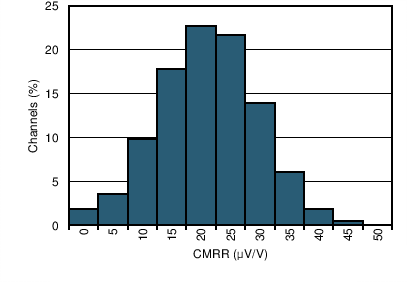5746 Channels VREF Pins Connected to Ground
Figure 1. Common-Mode Rejection Ratio Distribution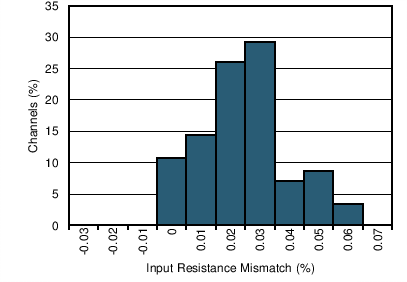5746 Channels
Figure 3. Distribution of Mismatch in 500-kΩ Input Resistors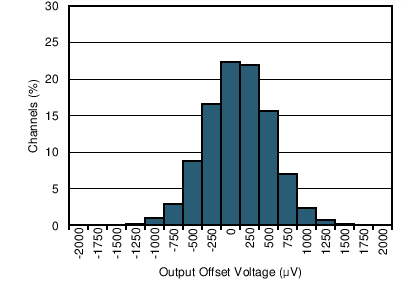5746 Channels
Figure 5. Offset Voltage Distribution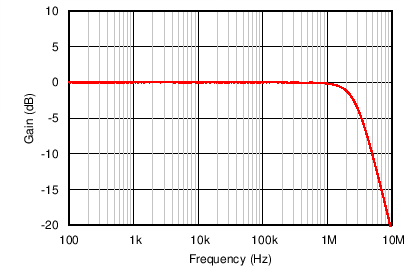Figure 7. Frequency Response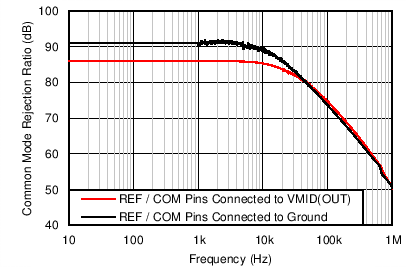Figure 9. Common-Mode Rejection Ratio vs Frequency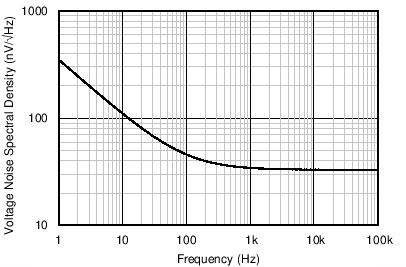Figure 11. Voltage Noise Spectral Density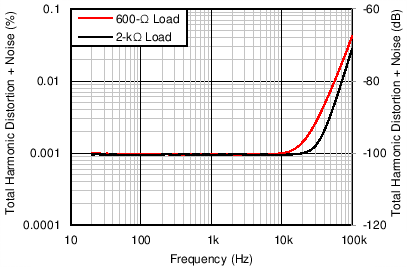3 VRMS, 500-kHz Measurement Bandwidth
Figure 13. THD+N vs Frequency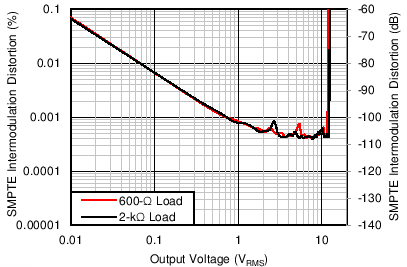SMPTE 4:1 60 Hz and 7 kHz, 90-kHz Measurement Bandwidth
Figure 15. SMPTE Intermodulation Distortion vs Output Amplitude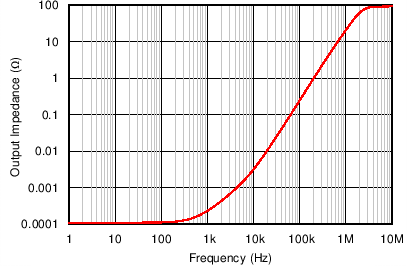Figure 17. Signal Path Output Impedance vs Frequency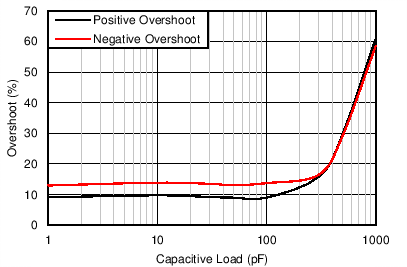100-mV Input Step
Figure 19. Overshoot vs Capacitive Load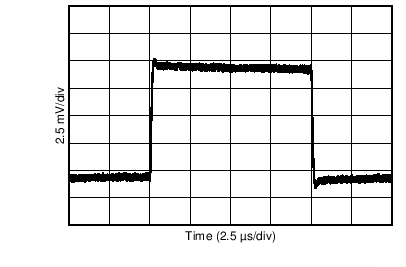10-mV Input Step
Figure 21. Small-Signal Step Response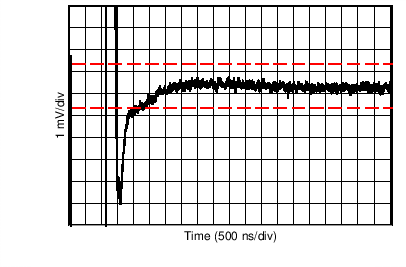10-V Input Step, 0.01% Settling = ± 1 mV
Figure 23. Rising-Edge Settling Time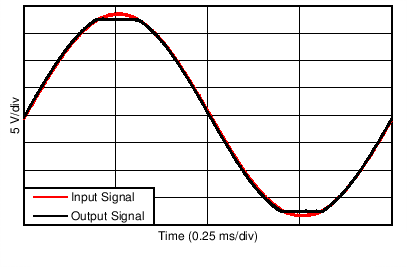Figure 25. No Phase Reversal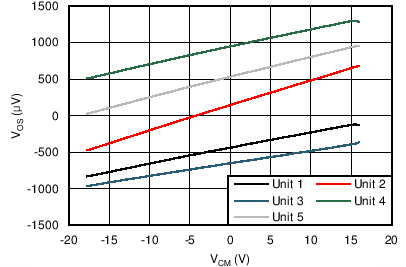5 Typical Units
Figure 27. Output Offset Voltage vs Common-Mode VoltageFigure 29. Positive Output Voltage vs Output Current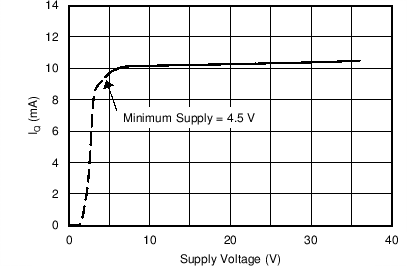Figure 31. Power Supply Current vs Power Supply Voltage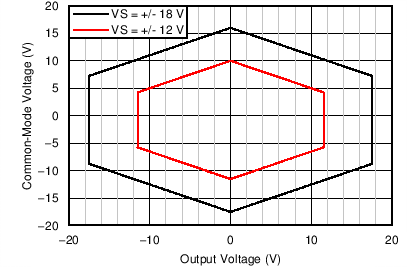REF A/B connected to 0 V
Figure 33. Input Common-Mode Voltage vs Output Voltage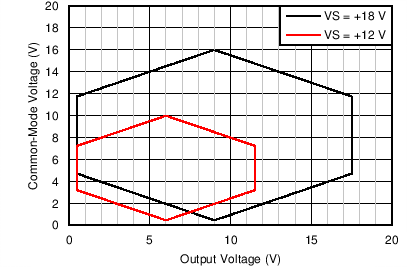REF A/B connected to VMID(OUT)
Figure 35. Input Common-Mode Voltage vs Output Voltage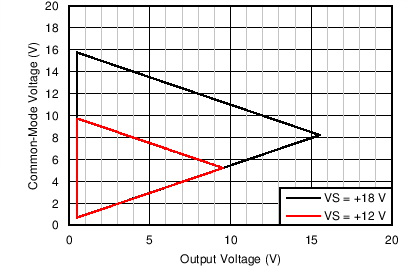REF A/B connected to 0 V
Figure 37. Input Common-Mode Voltage vs Output Voltage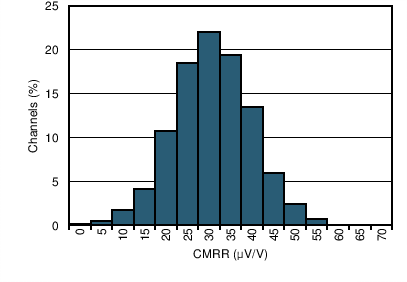5746 Channels VREF Pins Connected to VMID(OUT)
Figure 2. Common-Mode Rejection Ratio Distribution5746 Channels
Figure 4. Gain Error Distribution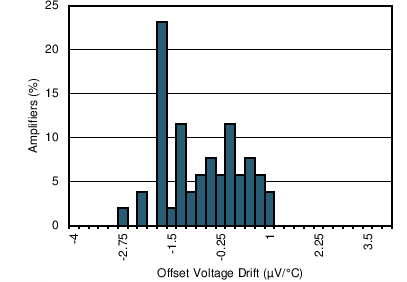52 Channels
Figure 6. Offset Voltage Drift Distribution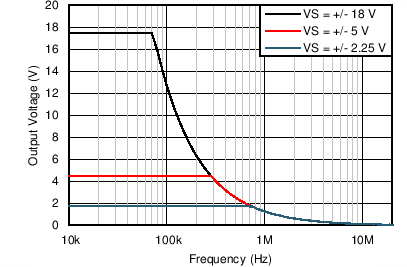Figure 8. Maximum Output Voltage vs Frequency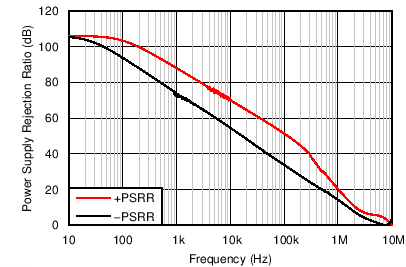Figure 10. Power Supply Rejection Ratio vs Frequency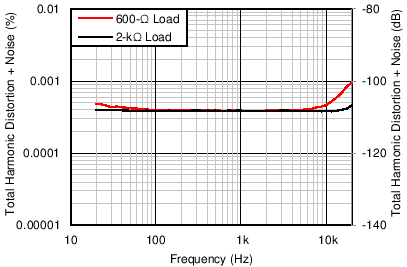3 VRMS, 90-kHz Measurement Bandwidth
Figure 12. THD+N vs Frequency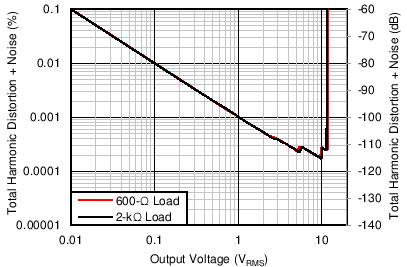1 kHz, 90-kHz Measurement Bandwidth
Figure 14. THD+N vs Output Amplitude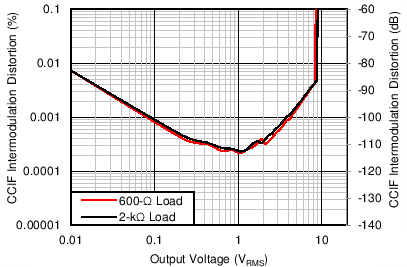CCIF 19 kHz and 20 kHz, 90-kHz Measurement Bandwidth
Figure 16. CCIF Intermodulation Distortion vs Output Amplitude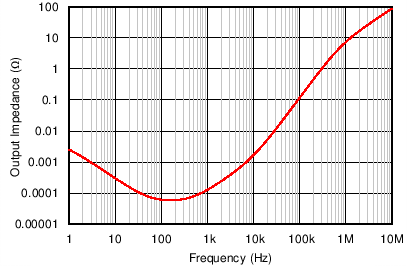CF = 1 µF
Figure 18. Supply Divider Output Impedance vs Frequency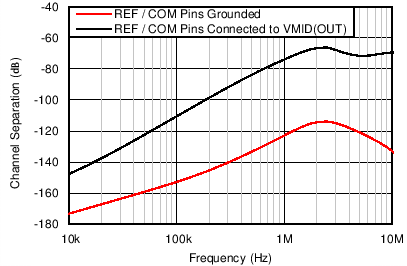Figure 20. Channel Separation vs Frequency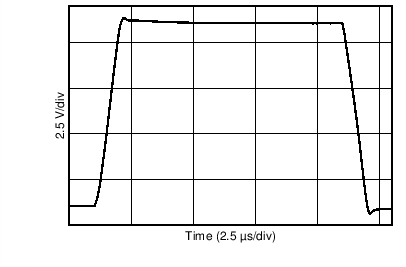10-V Input Step
Figure 22. Large-Signal Step Response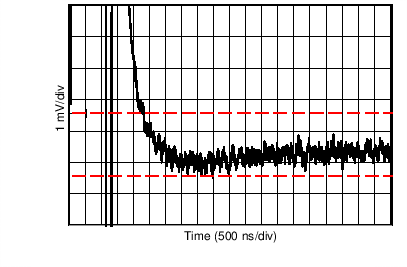10-V Input Step, 0.01% Settling = ± 1 mV
Figure 24. Falling-Edge Settling Time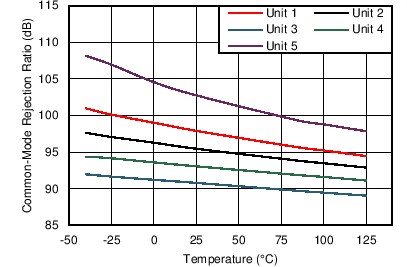5 Typical Units
Figure 26. CMRR vs TemperatureFigure 28. Short-Circuit Current vs Temperature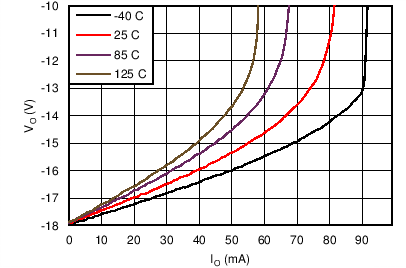Figure 30. Negative Output Voltage vs Output Current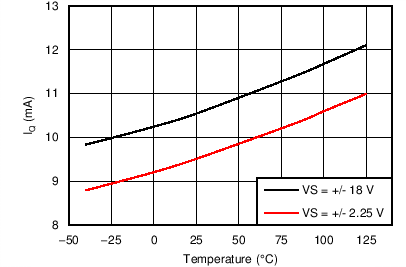Figure 32. Power Supply Current vs Temperature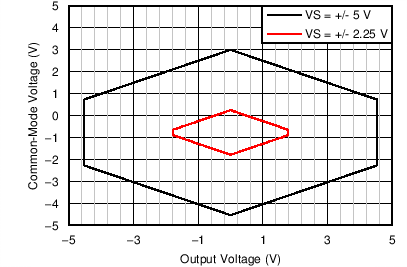REF A/B connected to 0 V
Figure 34. Input Common-Mode Voltage vs Output Voltage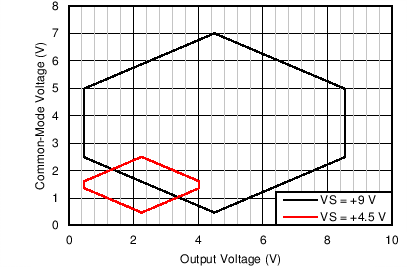REF A/B connected to VMID(OUT)
Figure 36. Input Common-Mode Voltage vs Output Voltage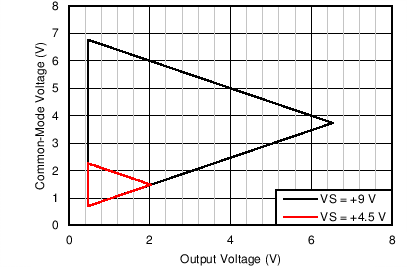REF A/B connected to 0 V
Figure 38. Input Common-Mode Voltage vs Output Voltage